Courses
Courses for Kids
Free study material
Free LIVE classes
More

# Area and Its Boundary Class 5 Notes CBSE Maths Chapter 11 (Free PDF Download)LIVE
Join Vedantu’s FREE Mastercalss

## Class 5 Maths Area and Its Boundary Revision Notes, Important Questions and NCERT Solutions

The Class 5 Chapter 11 Area and its Boundary revision notes are available on Vedantu to help students understand the topics covered in this chapter. From Class 5 Maths area and its boundary, students will learn the concepts of areas and the boundaries of different shapes such as squares and rectangles.

After going through our revision notes, students will easily be able to grasp the topics and form a clear understanding of the explanations without any difficulty. Our experts have used simple language and interactive exercises to make the learning process smoother for students. With the help of Class 5 Maths area and its boundary revision notes, students will be able to figure out which shape is bigger and how to measure the area of a shape just by simple calculations.

## Students can download the Class 5 Maths revision notes with the help of links provided below. These notes are created with the objective of making the learning process easier for students. You can make use of these notes for clearing your doubts, summarising the concepts and for practising important questions.

Last updated date: 01st Oct 2023
Total views: 145.2k
Views today: 2.45k

## What is a 2D figure?

• A two-dimensional shape is a plane figure that can be drawn on a flat surface.

• It has only two dimensions which are length and width. It has no thickness or depth

• Example: Rectangle, Circle, Square, etc

## What is Area?

• The space enclosed by a plane figure is referred to as its area (2 Dimensional shapes).

• Square units are used to measure area.

• The area of a plane figure is the number of squares required to completely cover it. Example: The area of the square given below will be the number of squares of the unit side required to completely cover the square.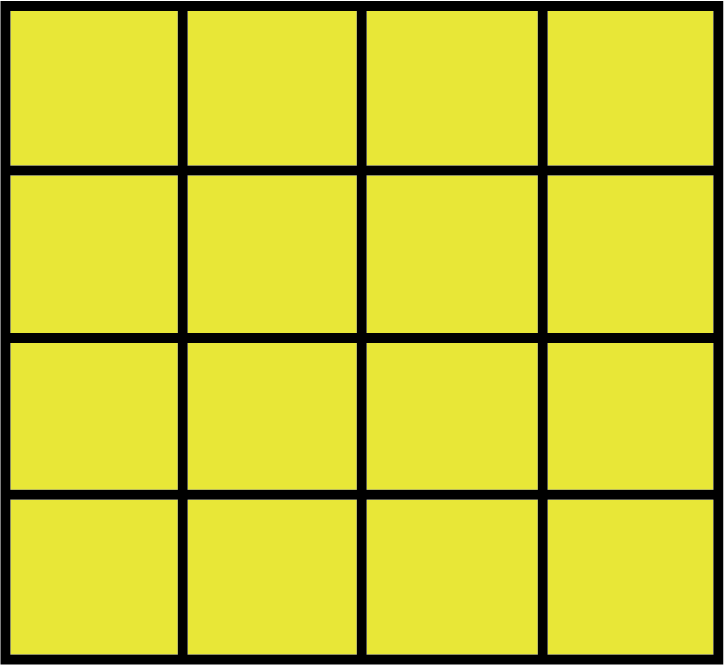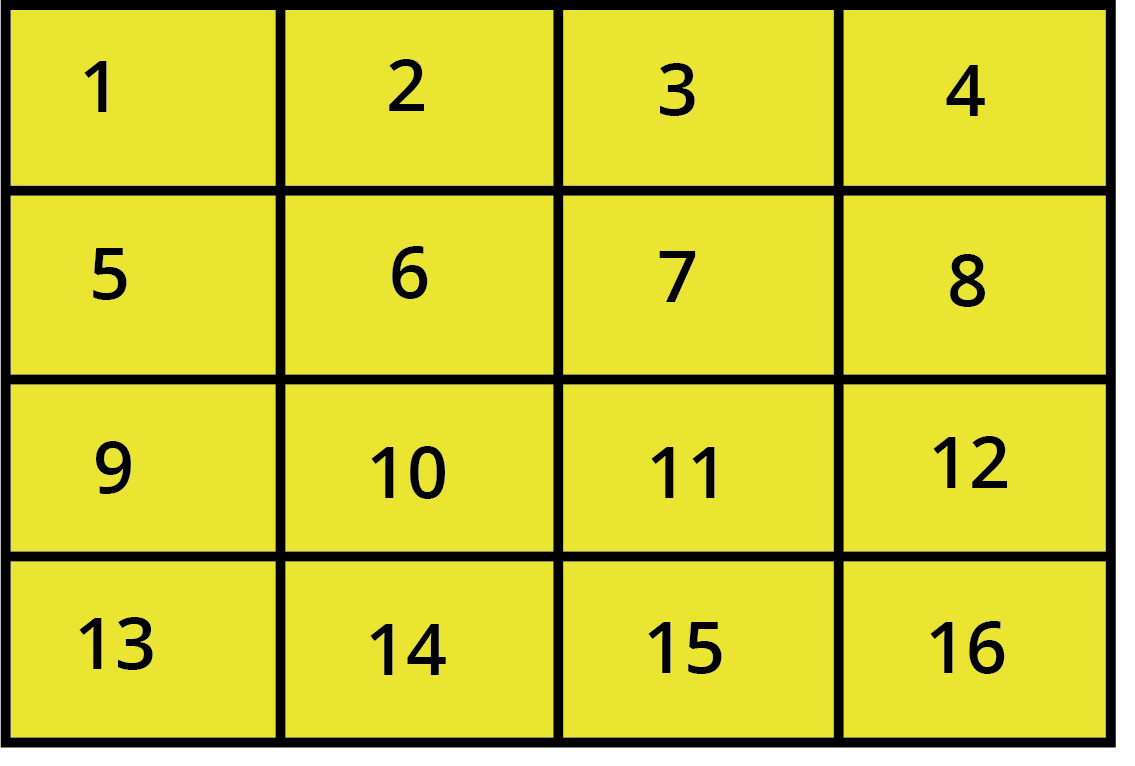Area of big Square

Since it would be silly to always count all those squares  to find the area       we just multiply both sides.

• Hence we can get formulae for the area as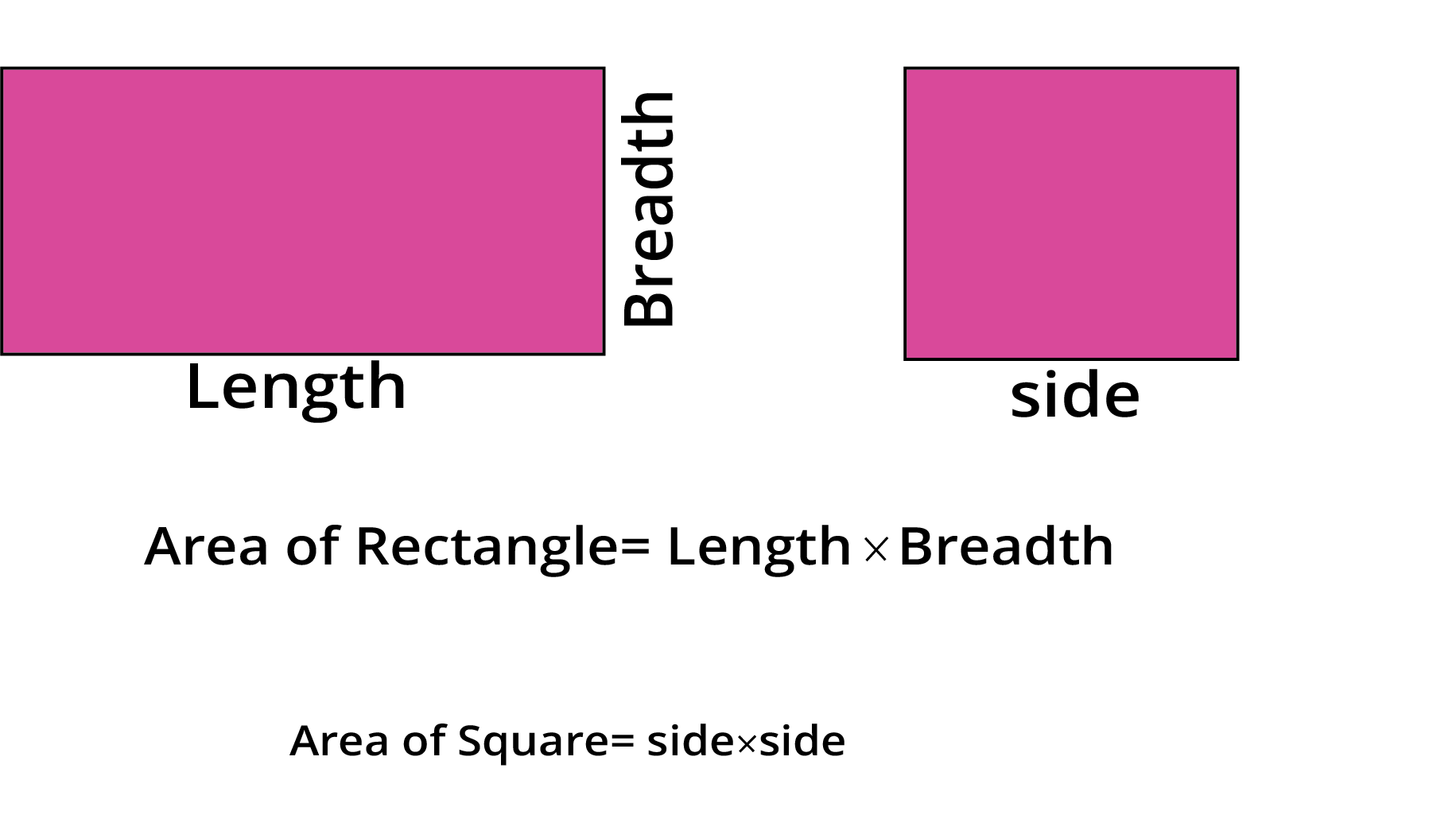Area of Rectangle and Square

• Formula to find the number of figures of area “x” to completely cover a figure of area “y” = y/x.

Example : No of pink sheets required to completely cover yellow sheet will be 18/3 = 6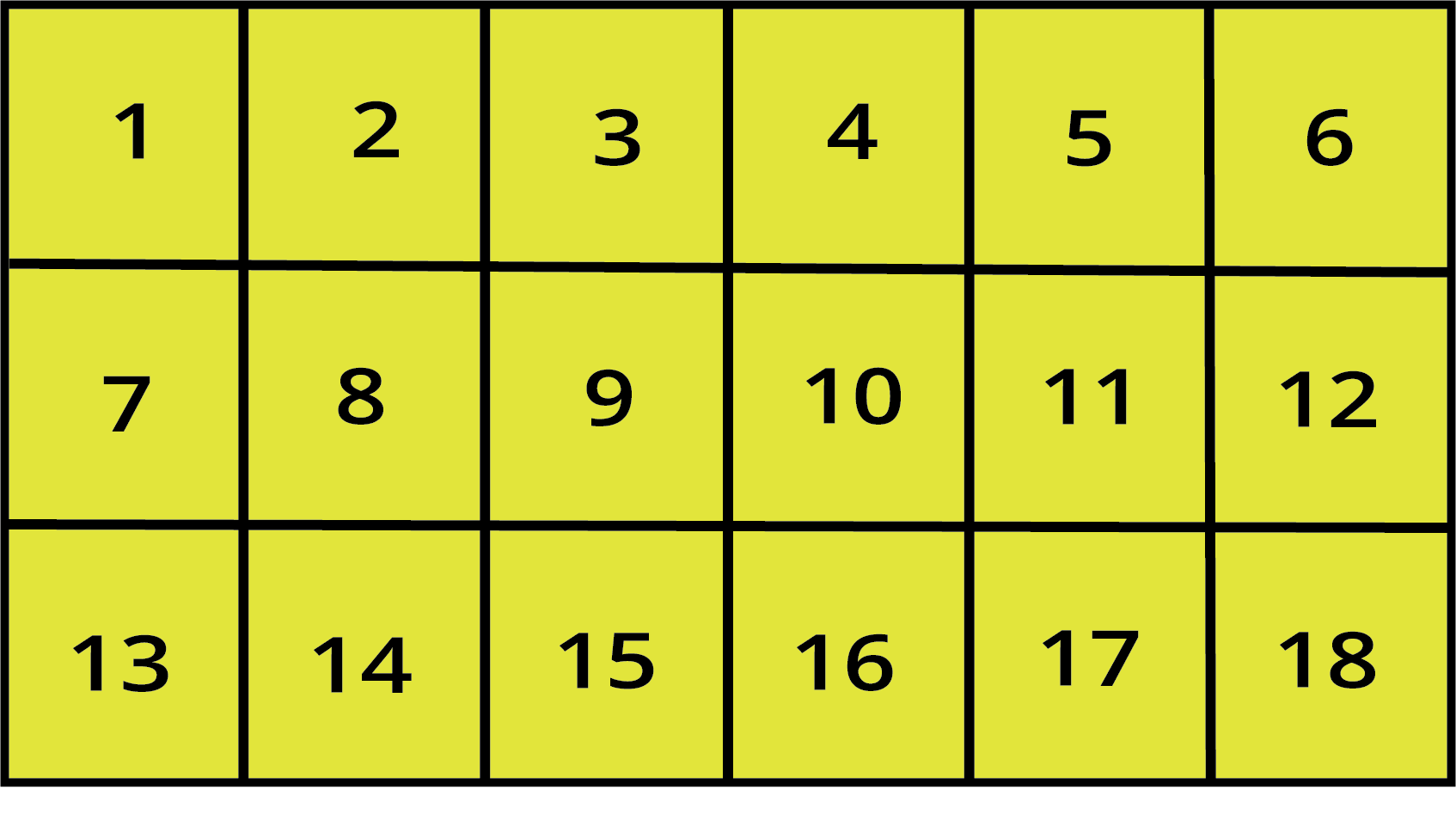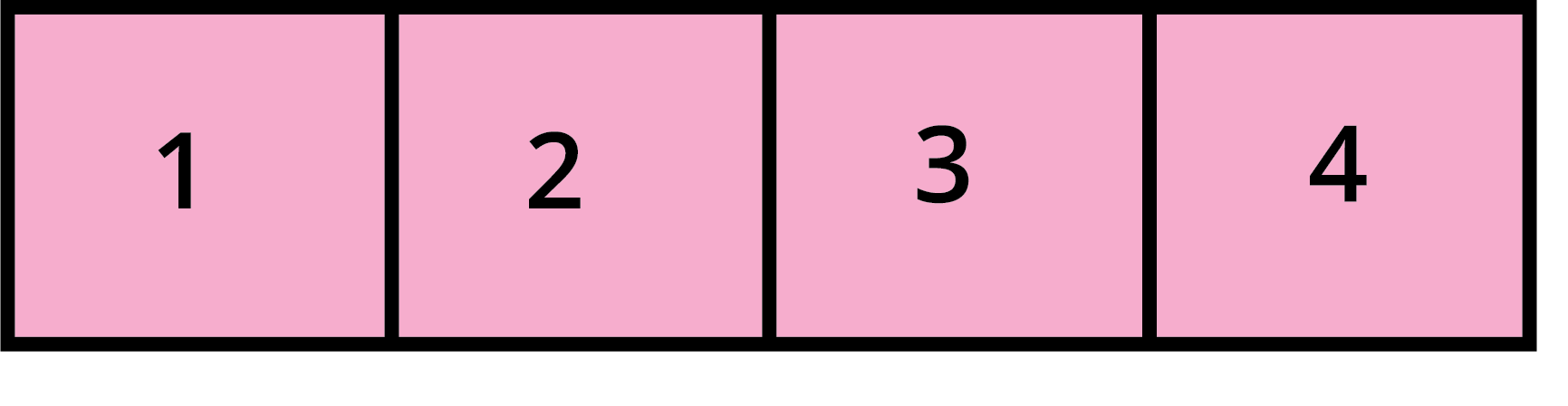Area of Rectangle

Class 5 Maths Area and Its Boundary Revision Notes [Solved Question]

Question 1. If the length of the board is 12 cm and the breadth is 10 cm and we want to cover the board with square sheets. The side of the square sheet is 2 cm. How many sheets are required to cover the board?

Solution: Length of board = 12 cm

Breath of board = 10 cm

Area of board = length x breadth

⇒ Area of board = (10 x 12) cm2

⇒ Area of board = 120 cm2

Side of sheet = 2 cm

Area of sheet =side × side

⇒ Area of sheet= (2 × 2) cm2

⇒ Area of sheet = 4 cm2

Number of sheets required = Area of board / Area of sheet

⇒ Number of sheets required = 120 / 4

⇒ Number of sheets required = 30

Answer. 30 sheets are required to cover the board.

## What is Perimeter?

• The perimeter of a two-dimensional figure is the length of the figure's boundary.

• If the figure is a triangle, square, or rectangle, the perimeter is the sum of the edge lengths.

• Example: Let’s find the perimeter of a given rectangle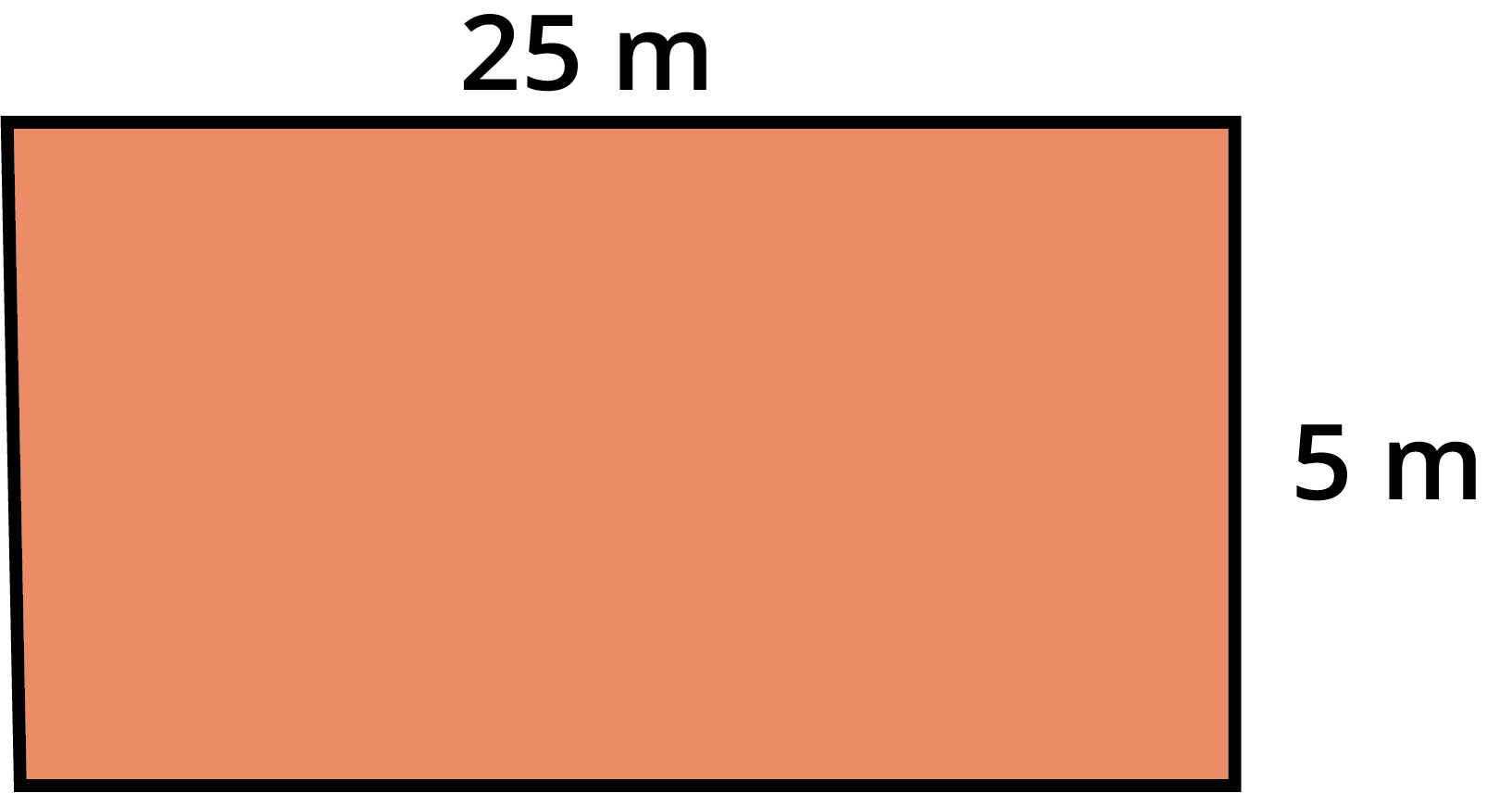Perimeter of Rectangle

Here the length of the rectangle is 25 m whereas the breadth of the rectangle is 5 m. Length of the boundary will be (25 + 5 + 25 + 5) m = 2(25 + 5)m = 60 m.

• Hence, we get formulae for perimeter as :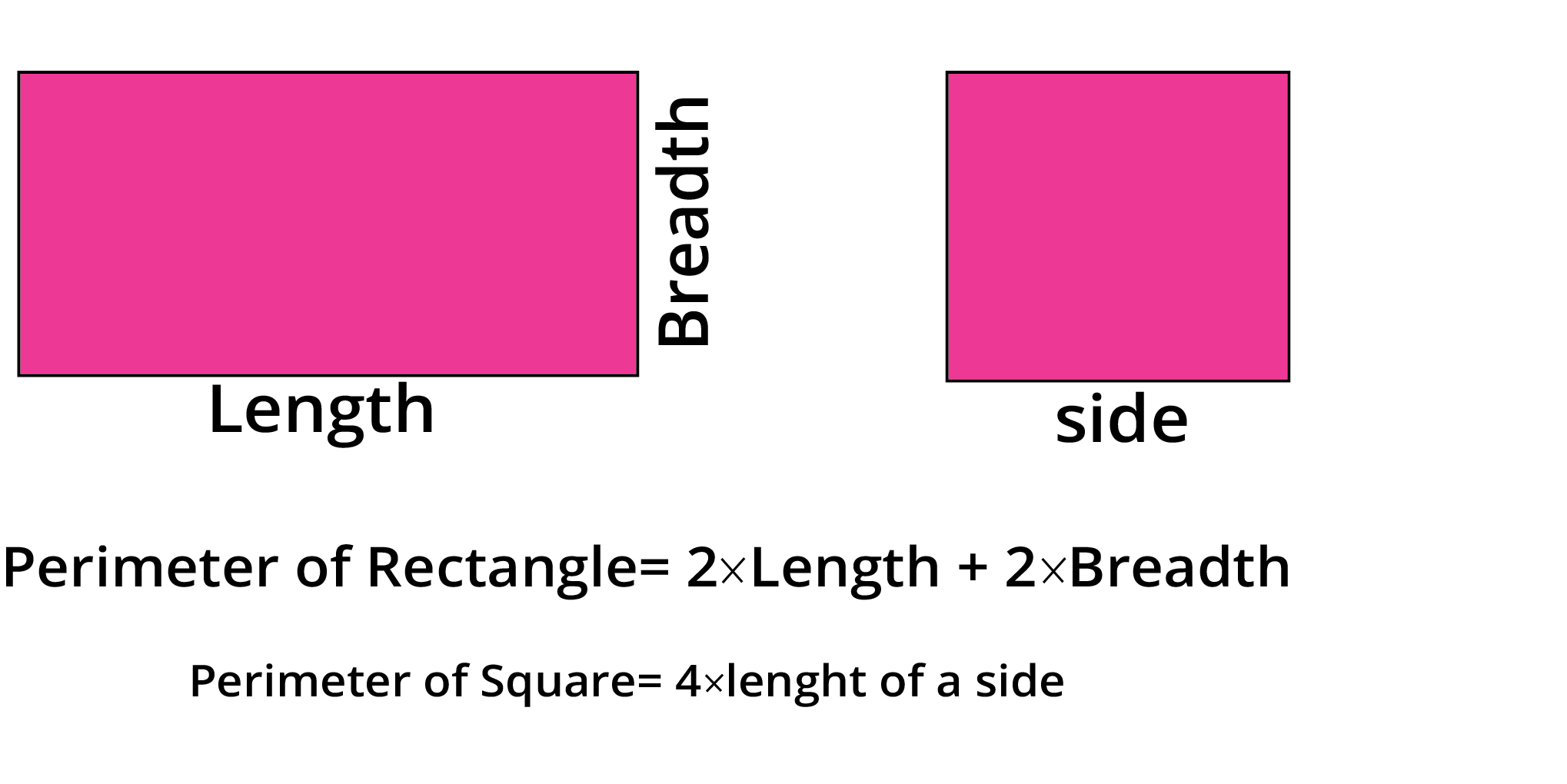Perimeter of Rectangle and Square

Question 2. It is known that the area of a square is 81 cm2. Find the perimeter of the square.

Solution: Area =81 cm2

Area = side × side

⇒ 81= (a)2

⇒ (9)2 = (a)2

⇒ 9 cm = a

⇒ Perimeter = 4 × side

⇒ Perimeter = 4 × 9

⇒ Perimeter = 36 cm

Answer. The perimeter of the given square is 36 cm.

### Units

• A unit of measurement is a definite magnitude of a quantity that is adopted by law and is used as a standard for measuring the same type of quantity.

• We measure the perimeter of any figure in mm, cm, m, or km.

• We measure the area of any figure in mm square, cm square, m square, or km square.

### Measurement Abbreviations and their comparisons

 Units Abbreviation Millimeter/millimeters mm Centimeter/centimeters cm Meter/meters m Kilometer/kilometers km Square millimetre mm2 Square centimetre cm2 Square meter m2 Square kilometre km2

1 mm < 1 cm < 1 m < 1 km

• 1 mm2 < 1 cm2 < 1 m2 < 1 km2

### Scale of drawing

• A drawing of a real object reduced or enlarged by a certain amount (called the scale).

• Example: A garden with a paved border is shown below. 1 cm on this garden is equal to 100 m on the ground.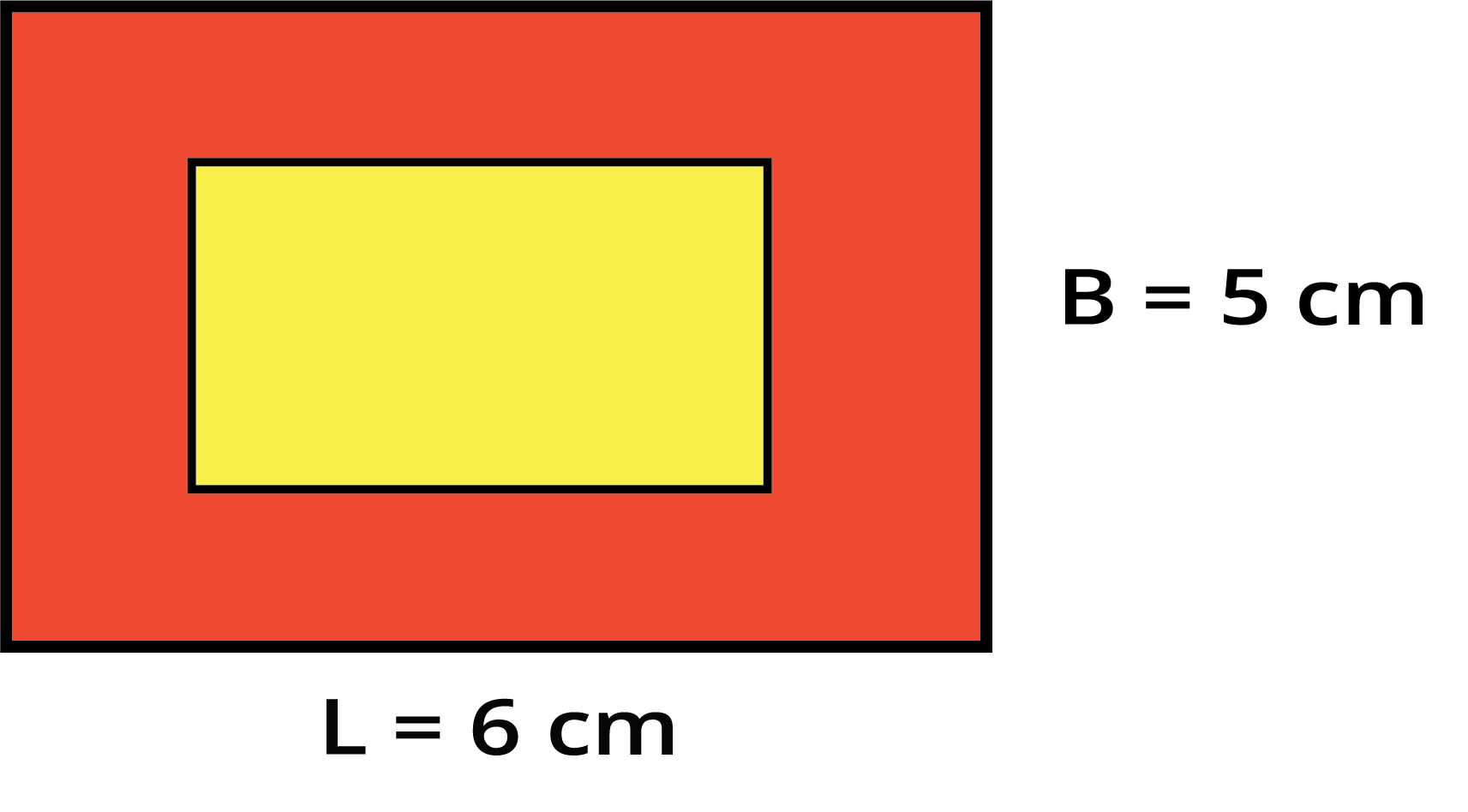Rectangular garden

Let’s find the length and breadth of the garden on the ground.

Length will be 6 x 100 m = 600 m.

Breadth will be 5 x 100 m = 500 m.

### Relationship between area and perimeter

• Consider two rectangles A and B having the same perimeter as 18 units.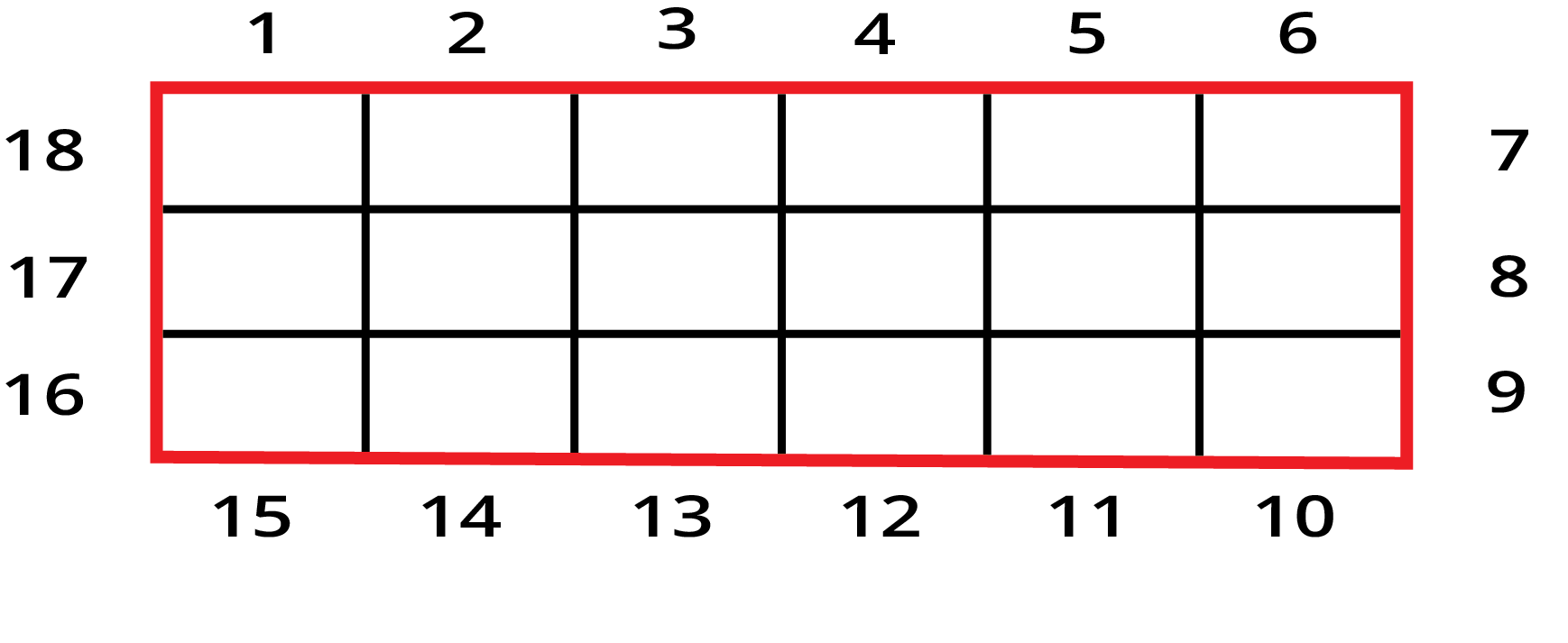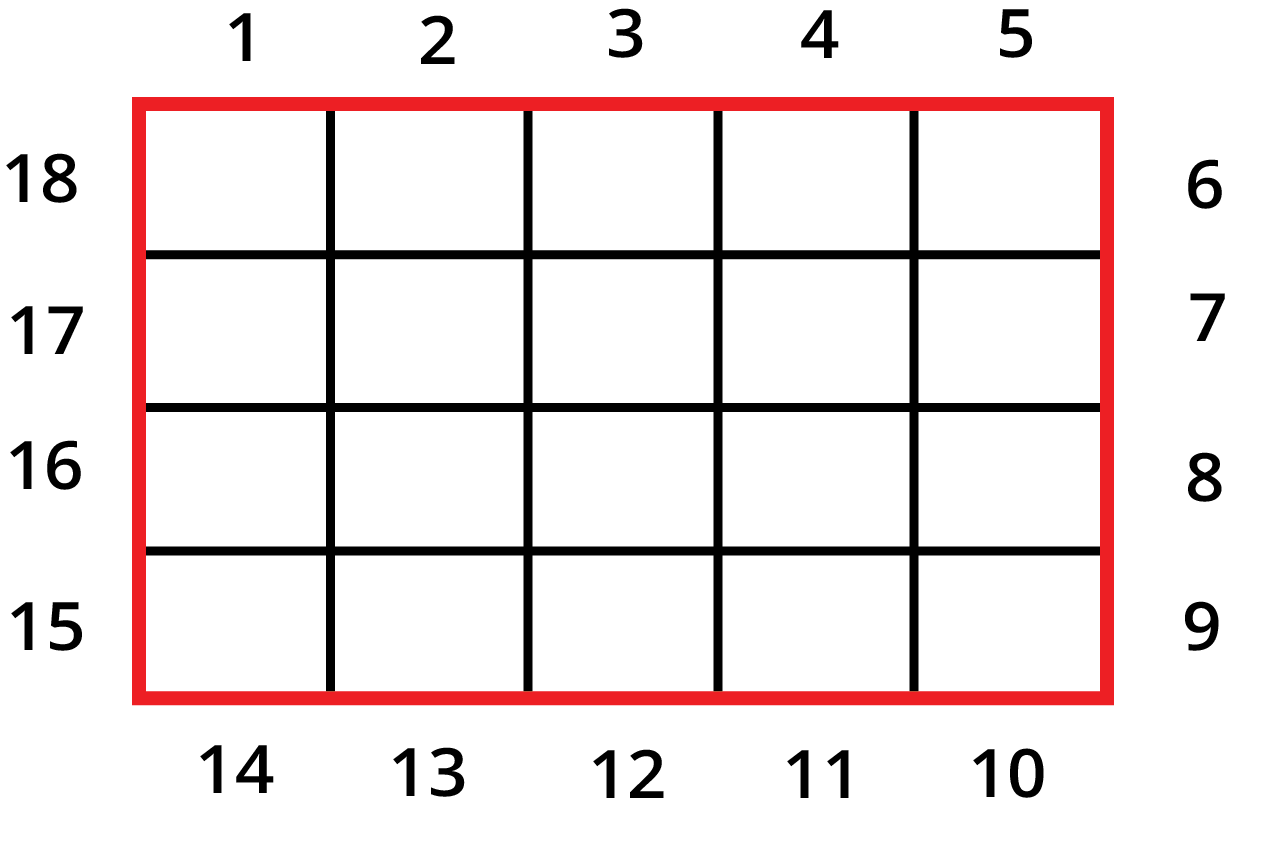Relationship between area and perimeter

Observe that both of them have different areas (Area of Rectangle A = 18 square units whereas Area of Rectangle B is 20 square units)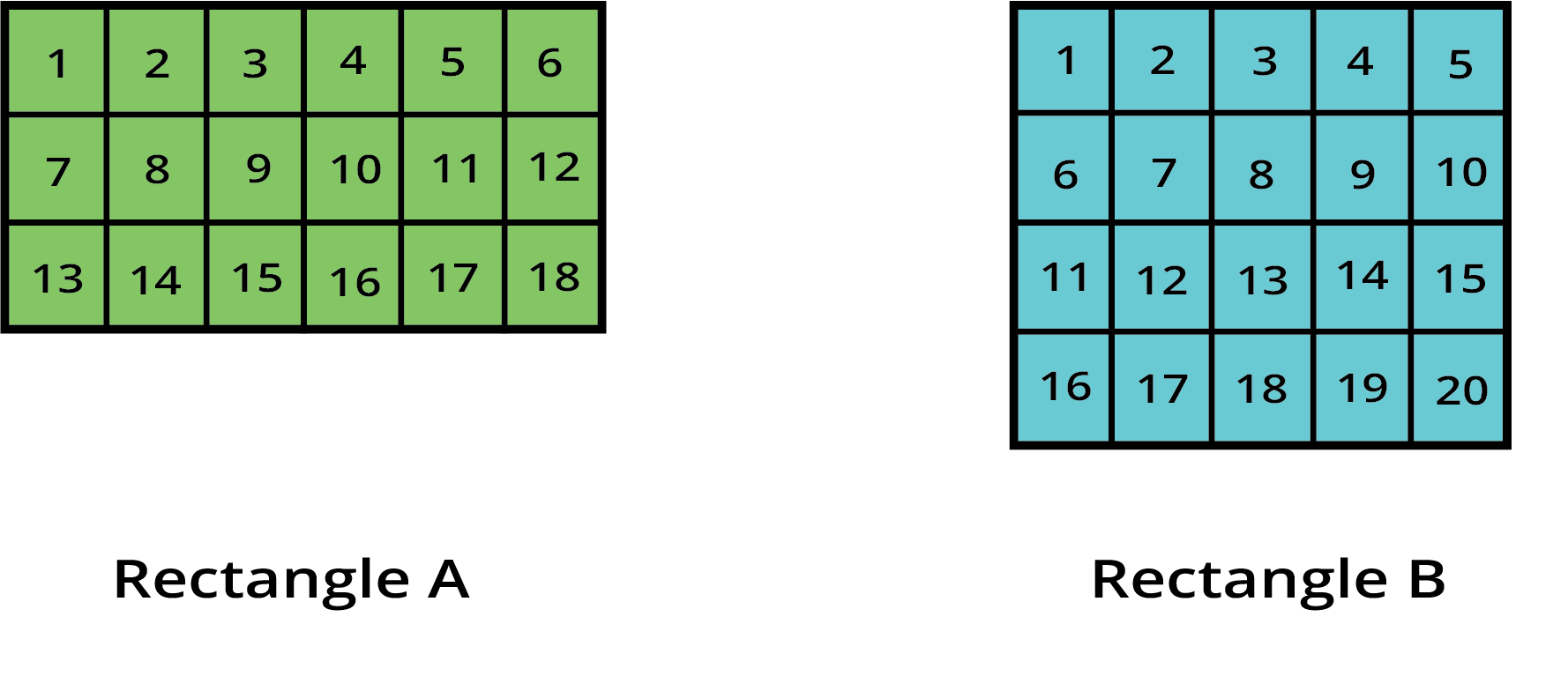Relationship between area and perimeter of a rectangle

Hence we conclude that:

•  The two shapes having the same perimeter can have different areas.

• Similarly, two shapes having the same areas can have different perimeters.

### Area when we cut image

• If we cut any 2D figure then the area of that 2D figure will be equal to the area of pieces. For example, if we cut a polygon into three pieces then the area of the polygon is equal to the sum of the area of three pieces.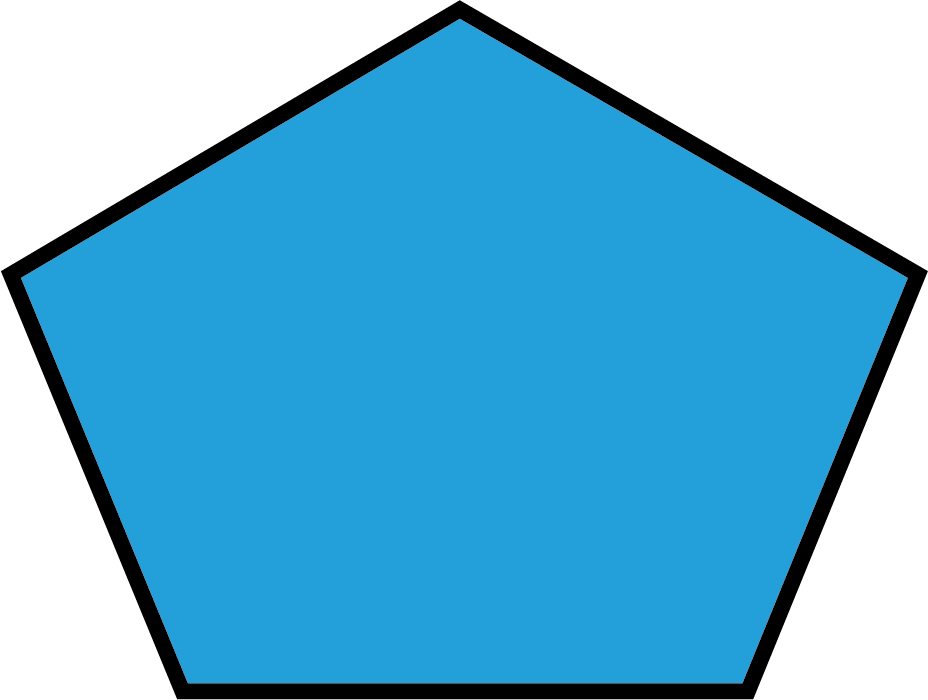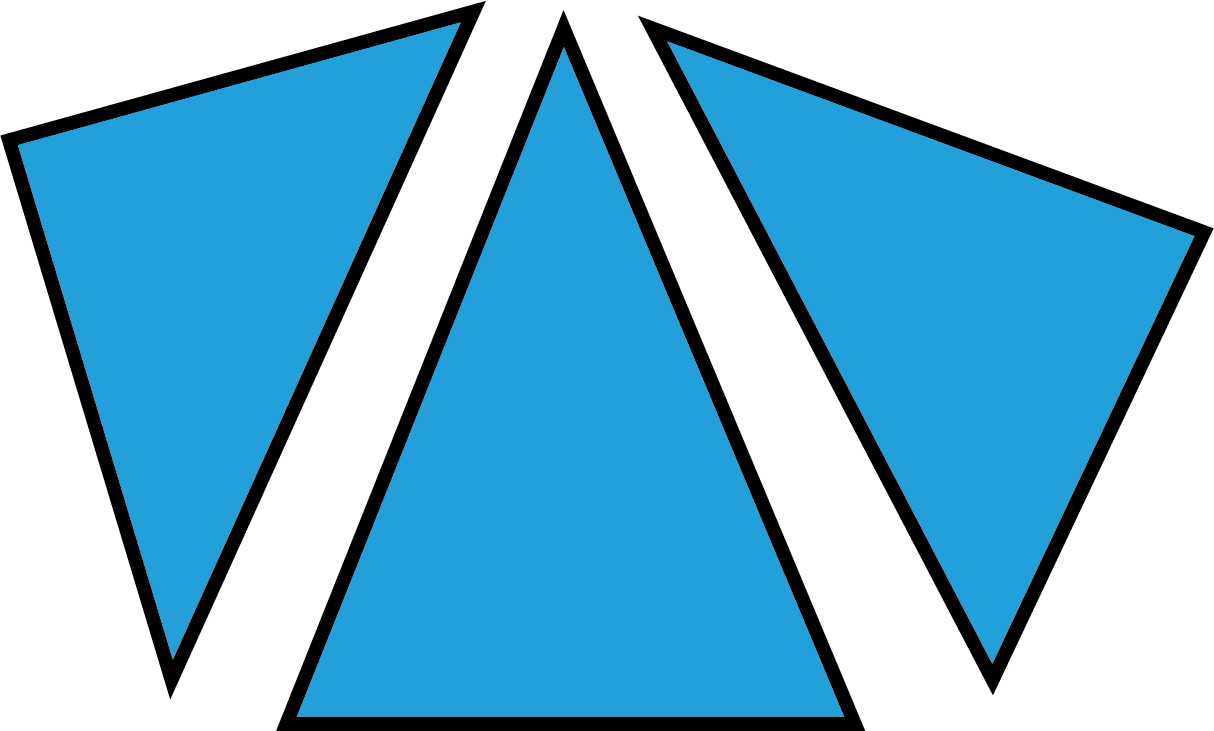Area when we cut the image

Question 3. Take a sheet of paper with a length of 14 cm and a breadth of 5 cm. Now cut this sheet into 5 equal rectangles. Find the area of each smaller rectangle.

Solution: Area of a sheet of length 14 cm and breadth 5 cm = Sum of the area of five equal rectangles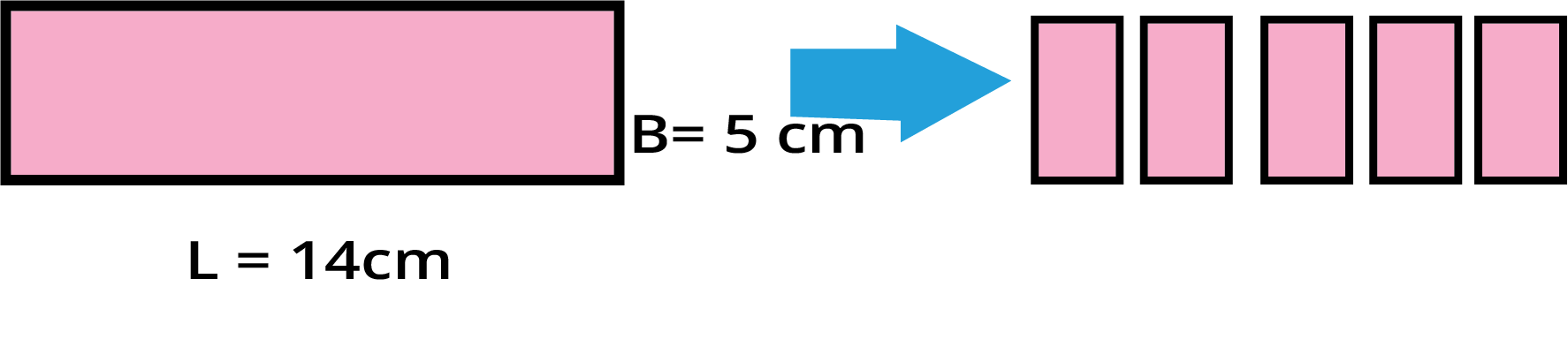Area of the big rectangle

Area of sheet of length 14 cm and breadth 5 cm = 5 x (area of small rectangle)

⇒ 14 x 5 = 5 x (area of small rectangle)

⇒ Area of small rectangle = (14 x 5) / 5

⇒ Area of small rectangle = 14 cm2

Answer. The area of each smaller rectangle is 14 cm2.

### Area of irregular shapes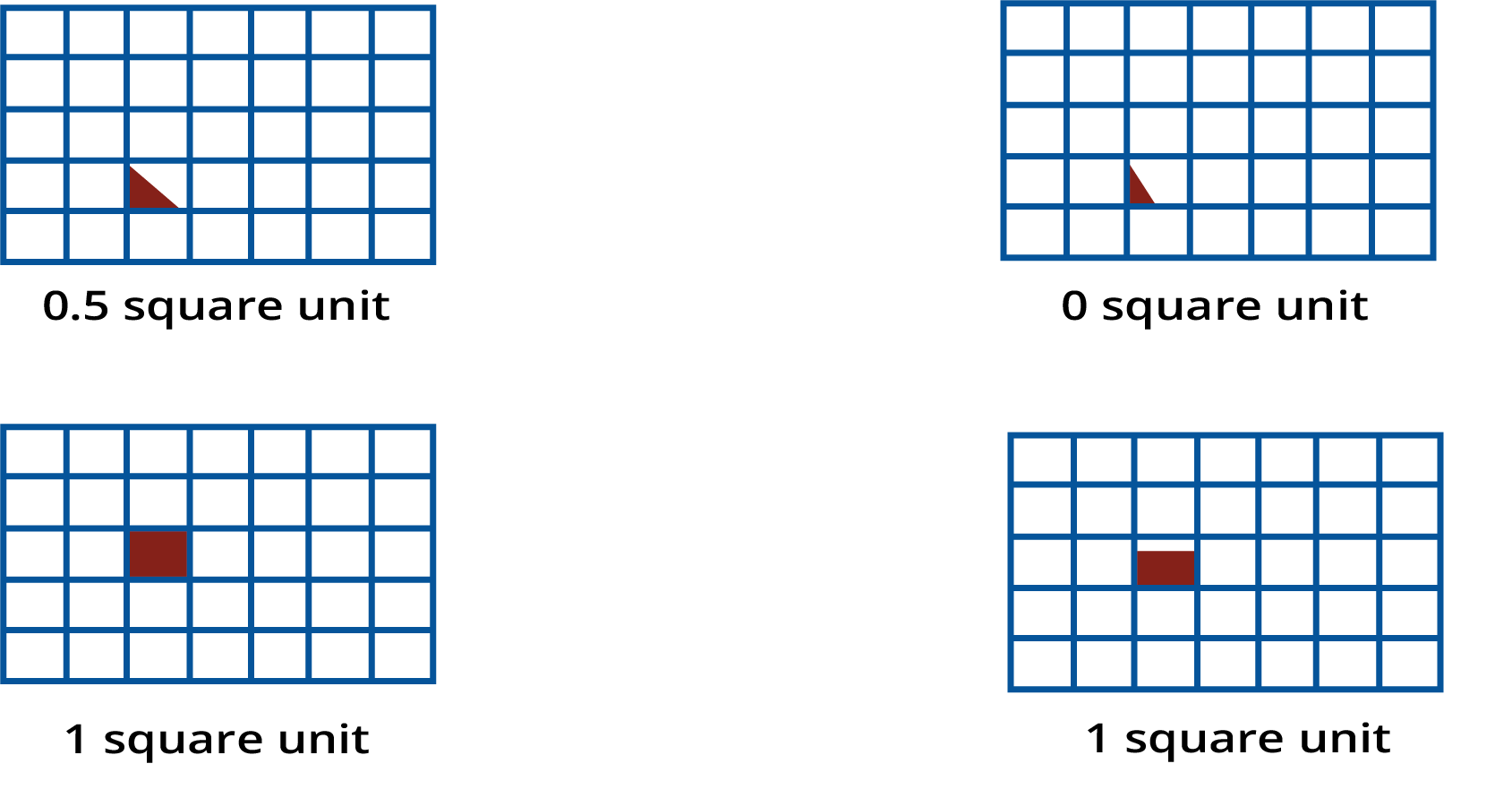Area of irregular shapes

Question 4. Find the area of the below figure: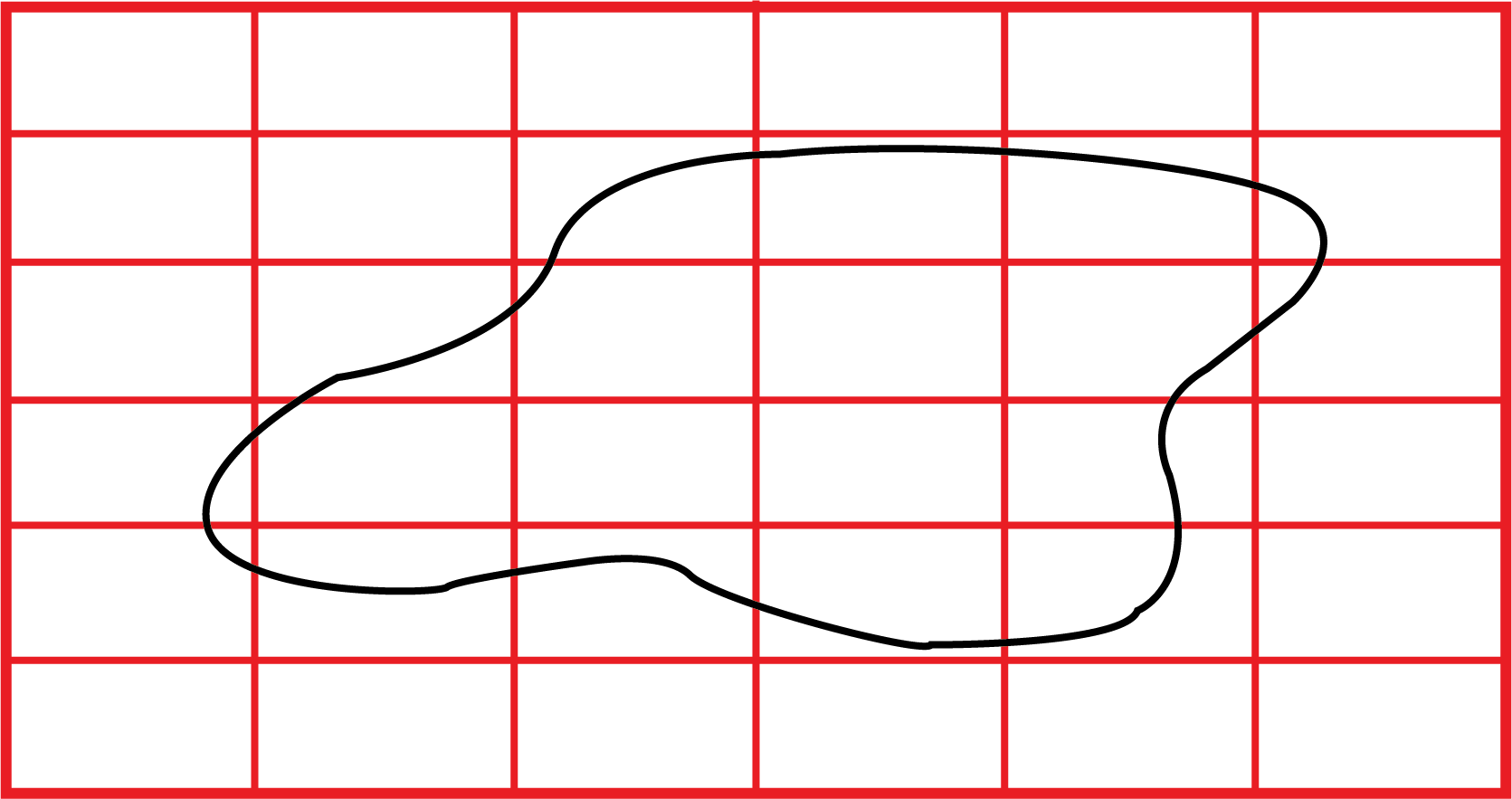Solution: After colouring and counting we observe that the total area of the figure will be (3+7+1) square units = 11 square units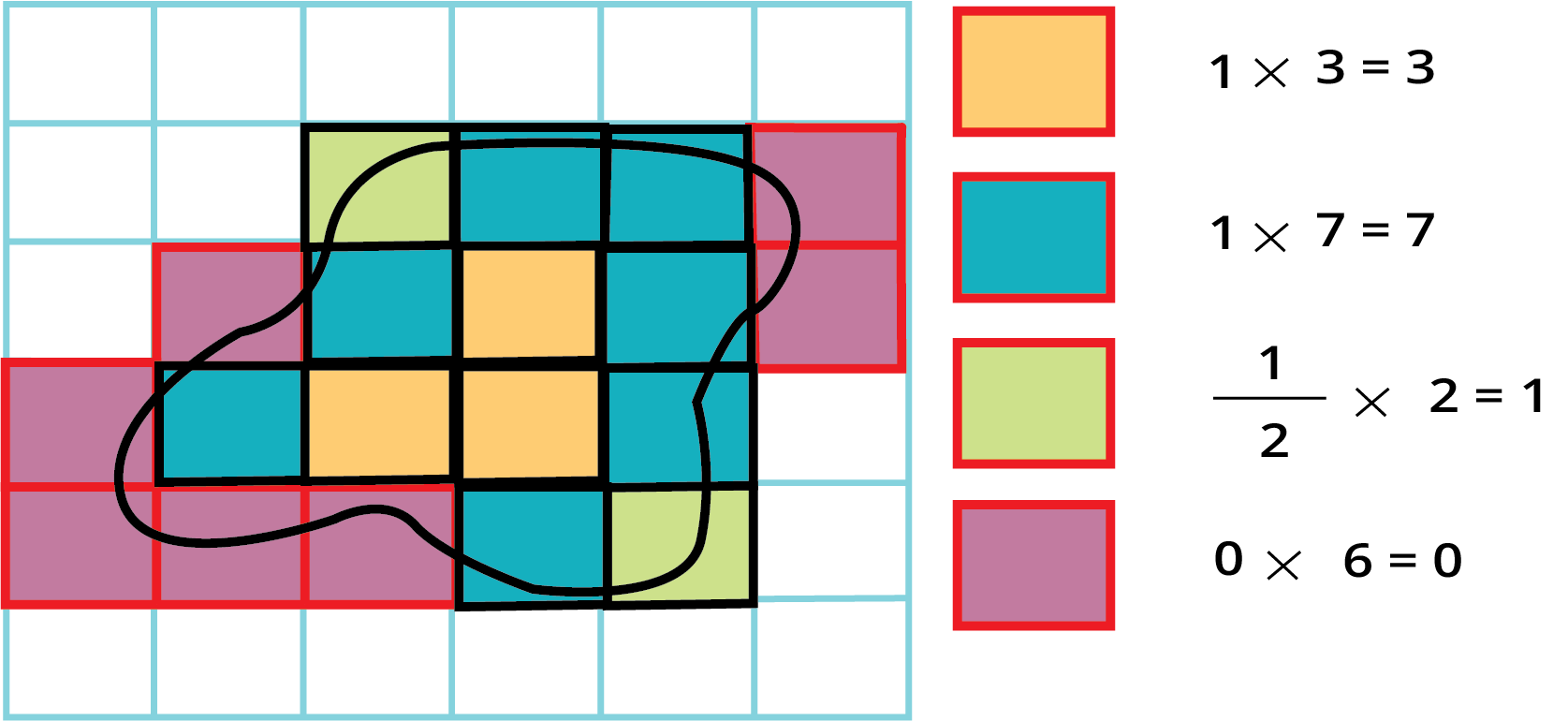### Practice Questions

Question 1. Umang plans to tile his kitchen floor with grey square tiles. Each side of the tile is 10 cm. His kitchen is 200 cm in length and 150 cm wide. How many tiles will he need?

Question 2. Rahul, Bhavika, and Kabir made rectangular greeting cards. Complete the table for their cards:

 Whose card Length Breadth Area Perimeter Rahul 5 cm 40 cm2 Bhavika 9 cm 3 cm Kabir 6 cm 60 cm

Question 3. Find the area of the below figure: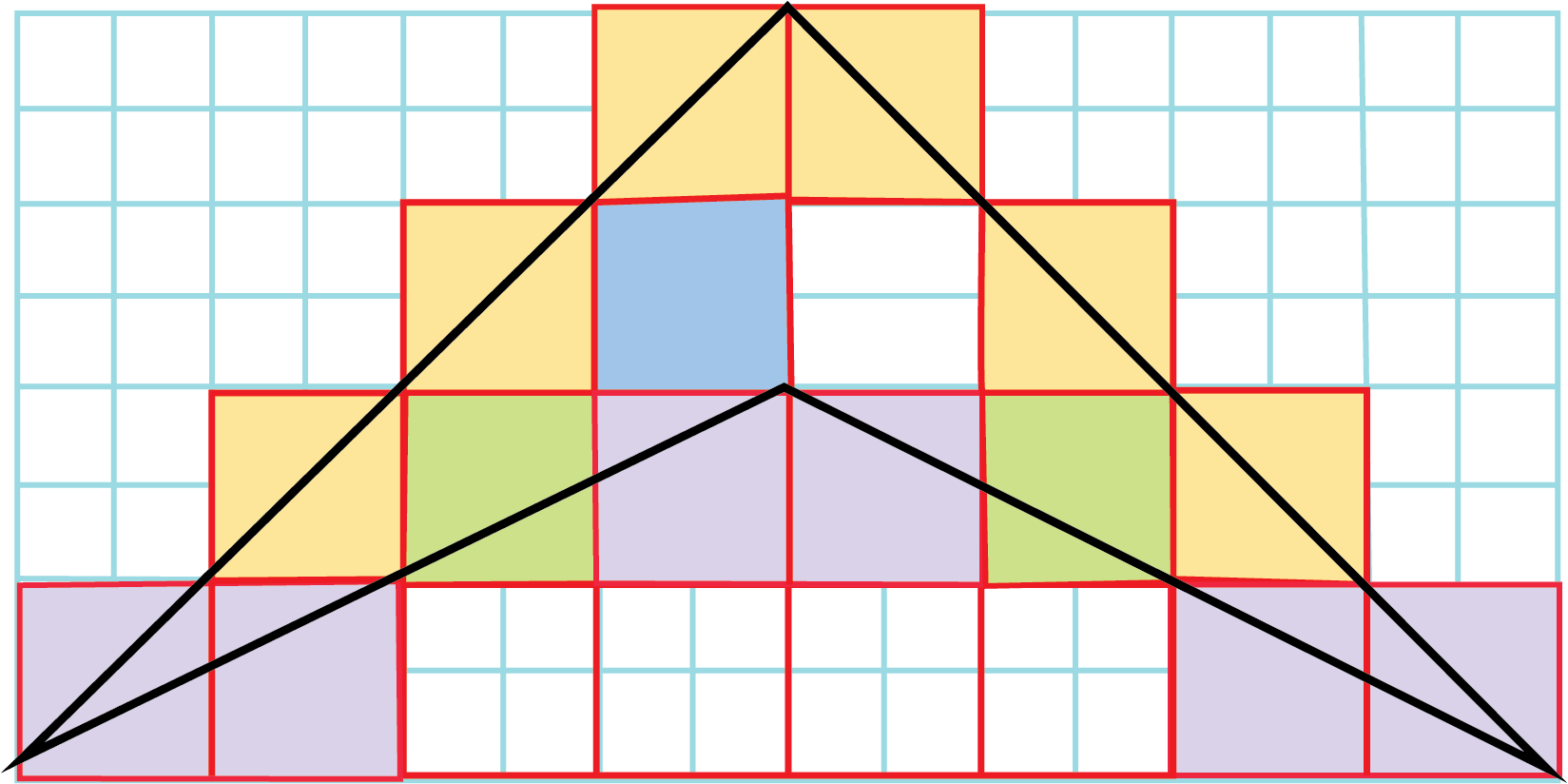Whose card Length Breadth Area Perimeter Rahul 5 cm 8 cm 40 cm2 26 cm Bhavika 9 cm 3 cm 27 cm2 24 cm Kabir 5 cm 10 cm 50 cm2 30 cm

Hint: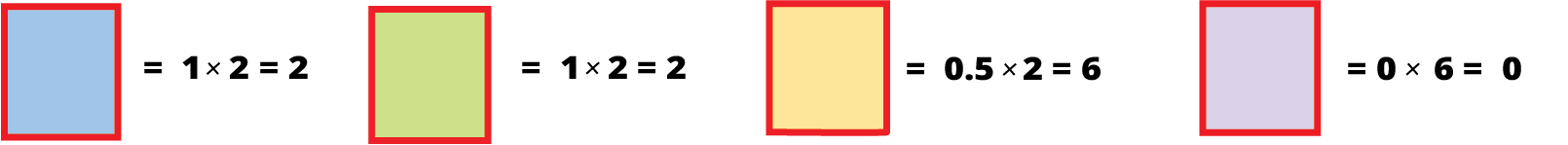## Class 5 Maths Area and Its Boundary Revision Notes [ Topic-list ]

Know the topics that are covered in the chapter- Area and Its Boundary. Practise all the topics mentioned below to have a complete preparation for your exams.

• Area and Perimeter

• Measure of Units

• Difference between smaller and larger shapes

• 2D Figures

• Measurement Abbreviations

• Scale of Drawing

• Relationship between Area and Perimeter

• Area of Cut Images

• Area of Irregular Shapes

## Class 5 Maths Area and Its Boundary Introduction

This chapter will primarily train students to distinguish between smaller and larger shapes on the basis of their area and dimensions. Further in this chapter you will learn the following:

• Applying formulas to calculate the area using measurements.

• Identifying patterns in 2D figures.

• Applying simple fractions to quantities.

• Clearing the concepts of area and perimeter.

• Determining the area of simple geometric figures.

## Importance of NCERT Maths Area and Its Boundary Class 5 Chapter 11

• The chapter will enable students to distinguish between the larger and smaller shapes on the basis of their area and dimensions.

• Students will get to learn different ways to compare the area of things which can help them in more complicated calculations in higher classes.

• This chapter prepares students for the advanced concepts of area, perimeter, and other related concepts that will have applications further during their academics.

• The detailed exercises and questions in the chapter will enable students to learn different problem-solving skills.

• Students will also be able to learn how to convert a square km to 1 square m and vice-versa in this chapter.

## Benefits of NCERT Maths Class 5 Chapter 11 Area and its Boundary Revision Notes

There are multiple benefits of opting to revise the chapter from the Area and Its Boundary Class 5 Worksheets with Solutions, and a few benefits are listed below.

• Getting a clear and precise understanding of how to find out the area of a figure such as a rectangle or square

• Using a simple approach to figure out how many different squares will it take to fill up a big rectangle

• A detailed explanation of different concepts can help students understand the chapter Area and its Boundary easily.

• Students will be able to complete the chapter easily and score well in the exams with the help of this important area and its boundary Class 5 explained notes.

All you need to do is thoroughly study the chapter and go through the revision notes and you will be able to solve even the toughest questions from the chapter. Solving these questions will also impact your problem-solving and time management abilities in the best way.

Download the revision notes for Area and its Boundary chapter that have been prepared by our experts at Vedantu for absolutely free. Gaining foundational knowledge about the area and boundaries of different shapes will become a lot easier with these notes.

## Preparation Tips for Class 5 Maths Area and Its Boundary

Our CBSE Class 5 Maths revision notes are free of cost and made by subject matter experts. You can read preparation tips provided by Vedantu for scoring higher marks in exams.

• Read the definitions of each topic and then learn the formulas related to that topic. For example: understand the concept of area of rectangles then memorise the formula of calculating area of rectangles.

• Once you have memorised the concept and formulas, practise the questions of NCERT books and also solve the important questions available online.

• Make sure to practise multiple questions on a regular basis so that solving problems doesn’t appear difficult anymore.

• Make a notebook just for noting down all the formulas and go through the listed formulas at least once daily.

• Include questions related to every geometrical figure present in NCERT textbook in your study schedule.

• Understand the difference between smaller and larger shapes with the help of explanation provided in revision notes.

## Conclusion

The Class 5 Maths area and its boundary revision notes available here are very helpful for students to prepare for their final exams. These notes and important questions adhere to the syllabus prescribed by CBSE for class 5. We are providing all the study materials such as NCERT solutions, revision notes, examples, and important questions. Students are encouraged to prepare for exams with the help of all reliable resources available online.

## FAQs on Area and Its Boundary Class 5 Notes CBSE Maths Chapter 11 (Free PDF Download)

1. How to convert a square km into 1 square m in chapter 11 of Class 5 Maths?

1km= 100m. So, 1 square kilometre will be (100 x 100) square metre. Thus, 1 square kilometre is equal to 10000 square metres. The aim of chapter 11 is to develop a sense of how big or small the units square kilometre and square metre are.

2. What does area of shapes mean chapter 11 of class 5 Maths?

The area of a shape refers to the space that encloses within the boundary of a specified shape. Thus, we calculate the area of several shapes by making use of the maths formulas.

3. Which Maths book is best for Class 5?

Here are some recommended books for class 5 Maths:

• Ratna Sagar Number Magic Mathematics Class 5

• Indiannica Learning Math Sight

• Think Maths!

• Together with Mathematics Buzz for Class 5

4. How can I figure out which shape is bigger in Chapter 11 Area and Its Boundary?

It is only after finding the area of the shape that you will be able to figure out which shape is the larger one. The Area and its boundary revision notes from Vedantu can help you with your exam preparation.

5. Is NCERT Class 5 Maths Chapter 11 important for the exam?

Yes, Class 5 Maths Chapter 11 Area and Its Boundary is a very important chapter and is essential from an exam point of view. Go through the revision notes and worksheets for this chapter as provided on Vedantu to practise the questions before the exam.